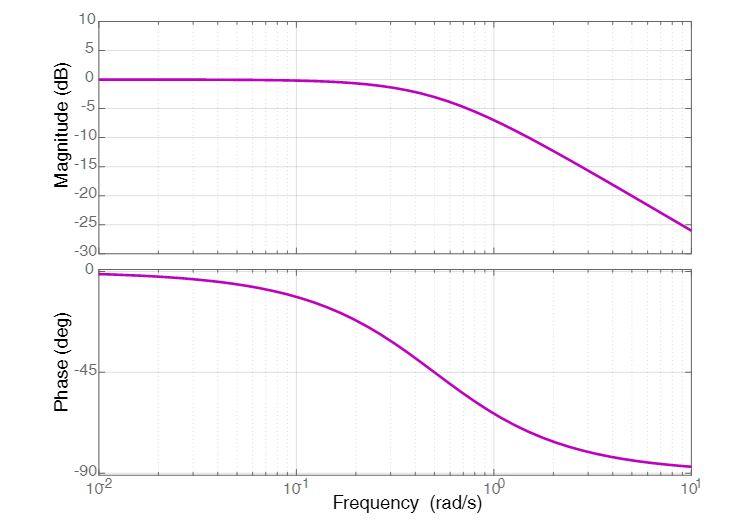Home / Control Systems / Bode Plot Example | Bode Diagram Example MATLAB

# Bode Plot Example | Bode Diagram Example MATLAB

Want create site? Find Free WordPress Themes and plugins.

## Bode Plot Example of First-Order System using Matlab

In this article, Bode Plot of Simple Phase-Lag Network (First Order System) is obtained using Matlab. In order to draw Bode Plot, we need transfer function from which we deduce the equations for Magnitude and Phase.

$G(s)=\frac{1}{2s+1}$

Function in the frequency domain can be written as:

$G(s)=\frac{1}{2j\omega +1}$

From above expression, we can deduce the corner frequency or break point as;

$\omega =\frac{1}{2}$

For Magnitude Plot:

When ω<<1 (very very small value), then

$G(s)\approx 1$

So, for very small value of ω, log magnitude of the transfer function would be;

$|G(j\omega ){{|}_{dB}}=20\text{ }log|G(j\omega )|=20\text{ }log(1)=0$

Hence, magnitude response would be constant below breakpoint.

When ω>>1 (very very large value), then

$G(s)\approx \frac{1}{2j\omega }$

So, for very large value of ω, log magnitude of the transfer function would be;

$|G(j\omega ){{|}_{dB}}=20\text{ }log|G(j\omega )|=20\text{ }log\left| \frac{1}{|2j\omega |} \right|=20\text{ }log\left( \frac{1}{2\omega } \right)=20log\left| 1 \right|-20log\left| 2\omega \right|=-20log(2\omega )$

So, above the break point, the magnitude plot would be a straight line with -20 dB/decade slopeNow, phase of the transfer function G(s) can be calculated as;

$\angle G(j\omega )=0-{{\tan }^{-1}}(\omega T)={{\tan }^{-1}}(\omega T)$

For Phase Plot:

When ω is very very small (ω≈0), then

$\angle G(j\omega )H(j\omega )={{0}^{\centerdot }}$

When ω is very very large (ω→∞), then

$\angle G(j\omega )H(j\omega )=-{{90}^{\centerdot }}$

Bode Plot Example Matlab Code

Here, we implemented the bode-plot for the comprehensive understanding of the readers.


% Bode Plot for Phase-Lag Network Example

clc

% Transfer function

K = ;

T = 2;

num = [K];

den = [T 1];

H = tf(num, den)

% Bode Plot

grid on

bode(H)

grid

%For Asymptotic Plot

% num=;

% den=[2 1];

% bode_asymptotic(num,den);



## Bode Plot for Phase-Lag Network ExampleDid you find apk for android? You can find new Free Android Games and apps.